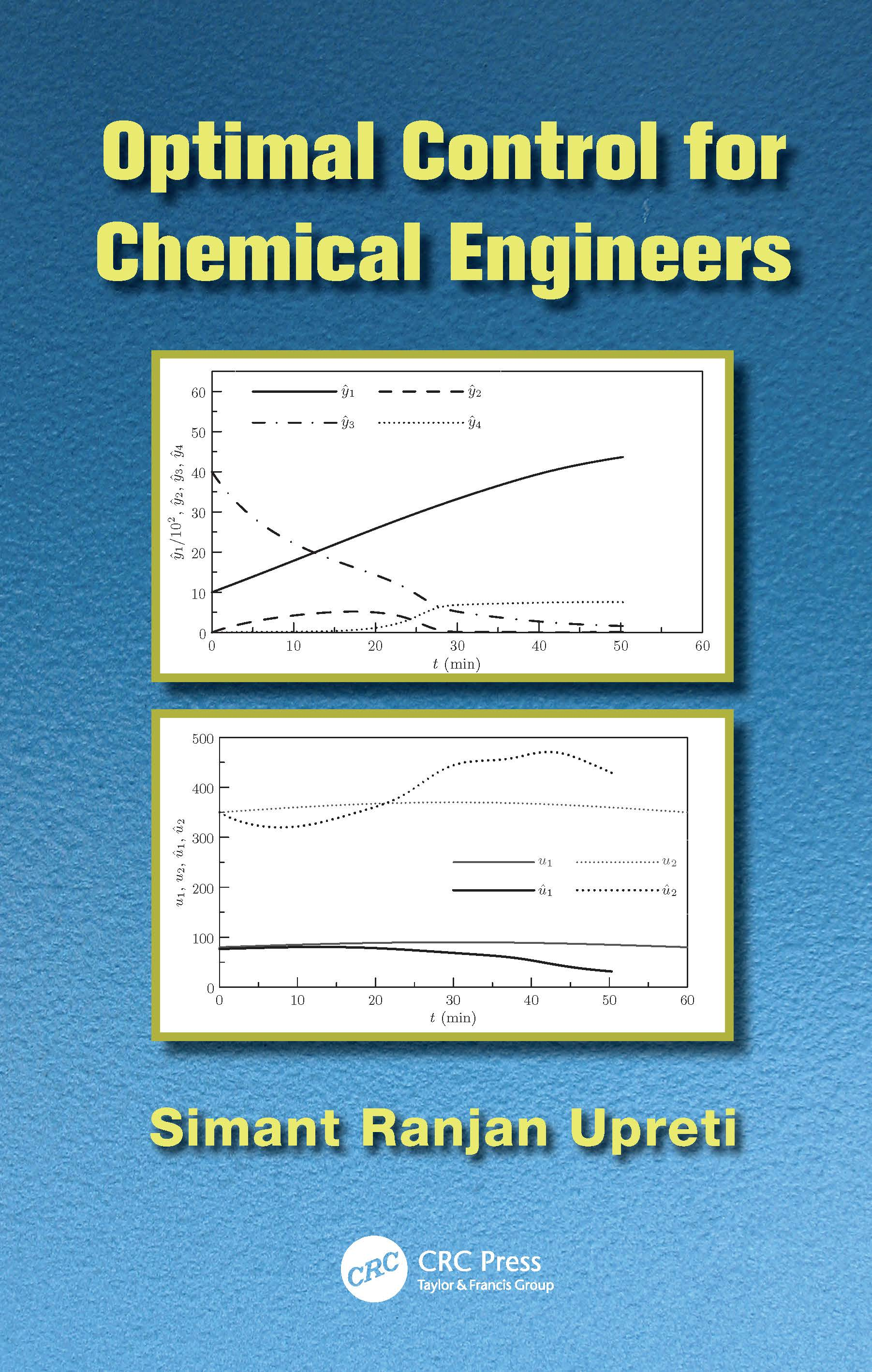Optimal Control for Chemical Engineers

1st Edition

CRC Press

305 pages | 79 B/W Illus.

Purchasing Options:\$ = USD
Paperback: 9781138074835
pub: 2017-03-30
SAVE ~\$17.39
\$86.95
\$69.56
x
Hardback: 9781439838945
pub: 2012-11-13
SAVE ~\$44.00
\$220.00
\$176.00
x
eBook (VitalSource) : 9780429094224
pub: 2016-04-19
from \$41.98

FREE Standard Shipping!

Description

Optimal Control for Chemical Engineers gives a detailed treatment of optimal control theory that enables readers to formulate and solve optimal control problems. With a strong emphasis on problem solving, the book provides all the necessary mathematical analyses and derivations of important results, including multiplier theorems and Pontryagin’s principle.

The text begins by introducing various examples of optimal control, such as batch distillation and chemotherapy, and the basic concepts of optimal control, including functionals and differentials. It then analyzes the notion of optimality, describes the ubiquitous Lagrange multipliers, and presents the celebrated Pontryagin principle of optimal control. Building on this foundation, the author examines different types of optimal control problems as well as the required conditions for optimality. He also describes important numerical methods and computational algorithms for solving a wide range of optimal control problems, including periodic processes.

Through its lucid development of optimal control theory and computational algorithms, this self-contained book shows readers how to solve a variety of optimal control problems.

Introduction

Definition

Optimal Control versus Optimization

Examples of Optimal Control Problems

Structure of Optimal Control Problems

Fundamental Concepts

From Function to Functional

Domain of a Functional

Properties of Functionals

Differential of a Functional

Variation of an Integral Objective Functional

Second Variation

Optimality in Optimal Control Problems

Necessary Condition for Optimality

Application to Simplest Optimal Control Problem

Solving an Optimal Control Problem

Sufficient Conditions

Piecewise Continuous Controls

Lagrange Multipliers

Motivation

Role of Lagrange Multipliers

Lagrange Multiplier Theorem

Lagrange Multiplier and Objective Functional

John Multiplier Theorem for Inequality Constraints

Pontryagin’s Minimum Principle

Application

Problem Statement

Pontryagin’s Minimum Principle

Derivation of Pontryagin’s Minimum Principle

Different Types of Optimal Control Problems

Free Final Time

Fixed Final Time

Algebraic Constraints

Integral Constraints

Interior Point Constraints

Discontinuous Controls

Multiple Integral Problems

Numerical Solution of Optimal Control Problems

Penalty Function Method

Shooting Newton-Raphson Method

Optimal Periodic Control

Optimality of Periodic Controls

Solution Methods

Pi Criterion

Pi Criterion with Control Constraints

Mathematical Review

Limit of a Function

Continuity of a Function

Intervals and Neighborhoods

Bounds

Order of Magnitude

Tayor Series and Remainder

Autonomous Differential Equations

Differential

Derivative

Newton-Raphson Method

Fundamental Theorem of Calculus

Mean Value Theorem

Intermediate Value Theorem

Implicit Function Theorem

Bolzano-Weierstrass Theorem

Weierstrass Theorem

Linear or Vector Space

Direction of a Vector

Parallelogram Identity

Triangle Inequality for Integrals

CauchySchwarz Inequality

Operator Inequality

Conditional Statement

Fundamental Matrix

Index

Bibliography and Exercises appear at the end of each chapter.